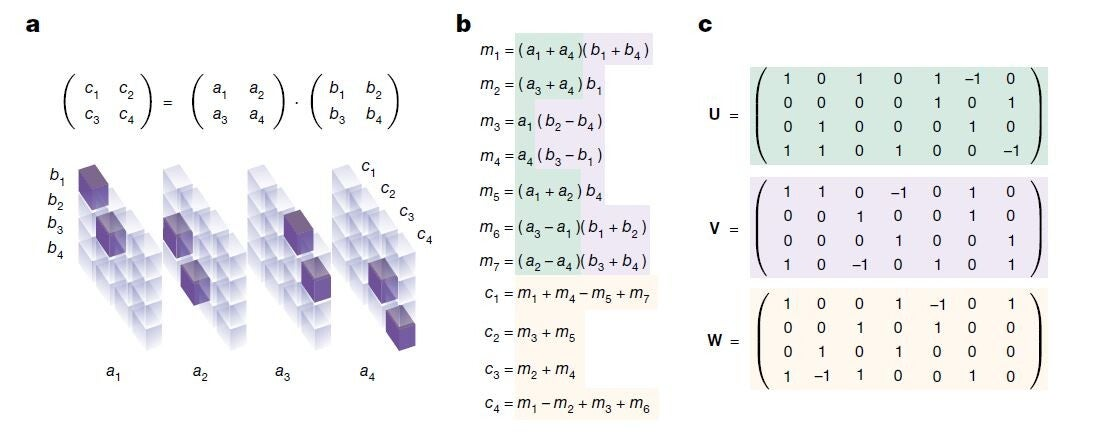# Discovering faster matrix multiplication algorithms with reinforcement learning

Published on 5 October 2022 at 21:04

### Alhussein Fawzi I DeepMind, London, UK I nature.com

Improving the efficiency of algorithms for fundamental computations can have a widespread impact, as it can affect the overall speed of a large amount of computations. Matrix multiplication is one such primitive task, occurring in many systems—from neural networks to scientific computing routines. The automatic discovery of algorithms using machine learning offers the prospect of reaching beyond human intuition and outperforming the current best human-designed algorithms. However, automating the algorithm discovery procedure is intricate, as the space of possible algorithms is enormous. Here we report a deep reinforcement learning approach based on AlphaZero1 for discovering efficient and provably correct algorithms for the multiplication of arbitrary matrices. Our agent, AlphaTensor, is trained to play a single-player game where the objective is finding tensor decomposition within a finite factor space. AlphaTensor discovered algorithms that outperform the state-of-the-art complexity for many matrix sizes. Particularly relevant is the case of 4 × 4 matrices in a finite field, where AlphaTensor’s algorithm improves on Strassen’s two-level algorithm for the first time, to our knowledge, since its discovery 50 years ago2. We further showcase the flexibility of AlphaTensor through different use-cases: algorithms with state-of-the-art complexity for structured matrix multiplication and improved practical efficiency by optimizing matrix multiplication for runtime on specific hardware. Our results highlight AlphaTensor’s ability to accelerate the process of algorithmic discovery on a range of problems, and to optimize for different criteria.